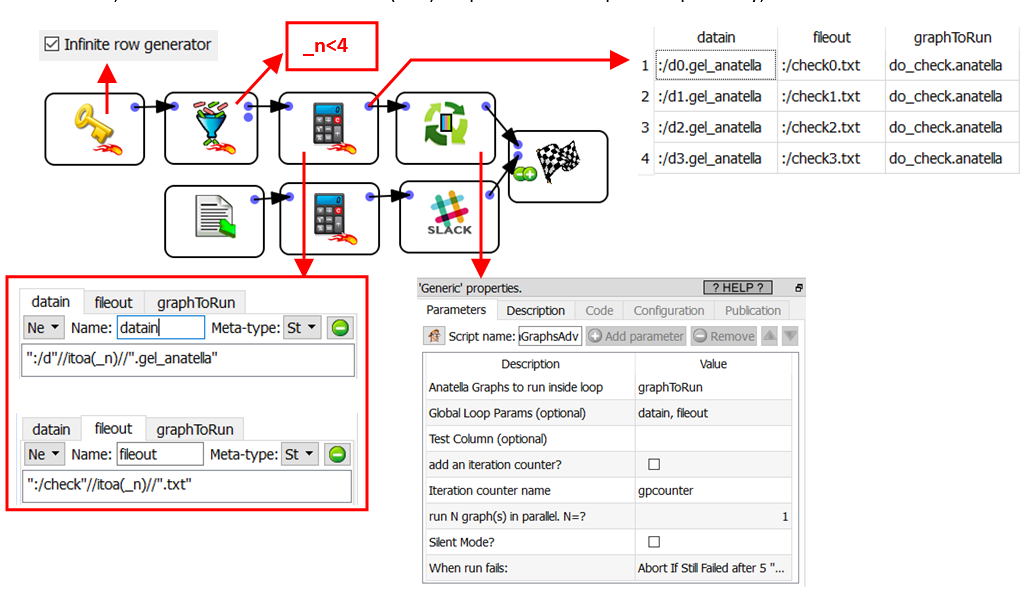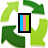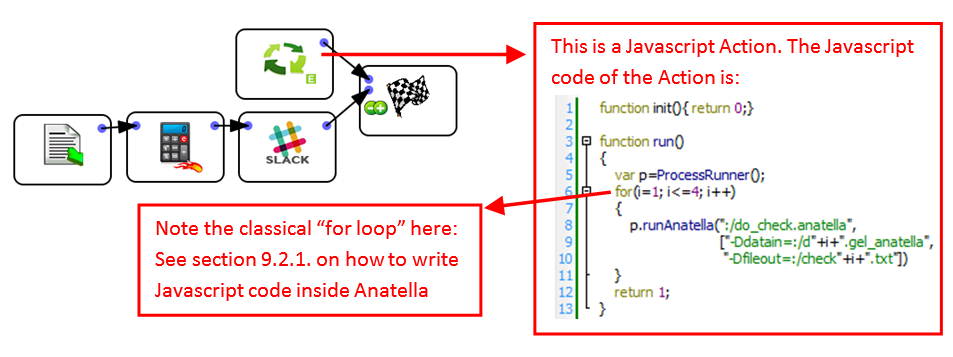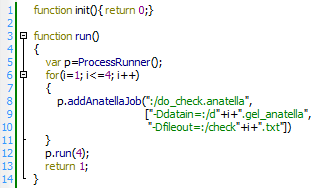﻿ 10. FAQ > 10.7. How to create “Loops” inside Anatella?

# 10.7. How to create “Loops” inside Anatella?

Let’s re-start from the example given in the previous section (i.e. the section 10.6).

In the example from the previous section, we only had 4 datafiles to check. When we have many files to check, we can embed the call to the (sub)Graphs inside a loop. More precisely, we’ll have:For another example of usage of theloopAnatellaGraphAdv action (to create “loops”), see the section 5.21.6.

If you prefer to code a little bit in Javascript, the following graph is equivalent to the above graph:In the above example (with the Javascript “Loop”), the 4 “checks” (i.e. the four subGraphs) are executed in a sequential order. If we want to execute the 4 graphs simultaneously (to divide the computation time by 4), we’d rather have the following Javascript code:You’ll find the documentation of the “ProcessRunner” Javascript Object inside the section 11.6.